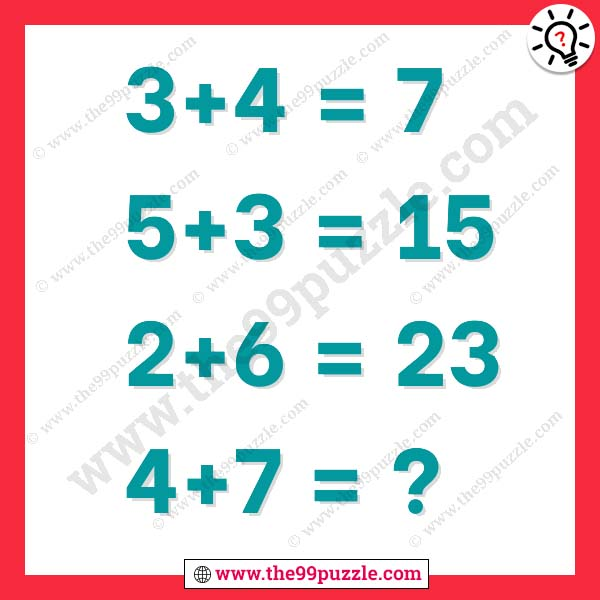# Mathematical riddle of logic question with answer – Puzz270

Test your math skills to solve this puzzle. Mathematical reasoning puzzles are asked in various exams. These types of puzzles are fun and logical. How good are you at math? Sometimes this math puzzle gets very hard but when you crack the math logic this is very easy to solve. In this mathematical riddle of logic question, some equations give here. All equations are in the same math logic. You have to crack the logic and solve the last equation.

3+4=7

5+3=15

2+6=23

4+7=?3+4=7

7+5+3=15

15+2+6=23

23+4+7=34